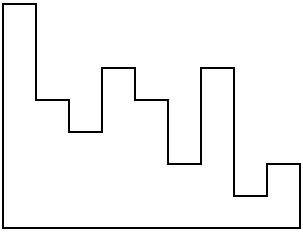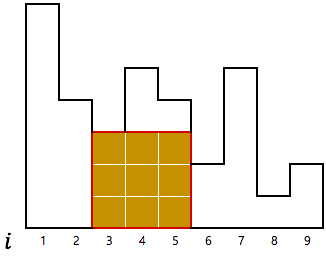시간 제한메모리 제한제출정답맞힌 사람정답 비율
2 초 (추가 시간 없음) 1024 MB138343127.928%

## 문제

A histogram is a polygon made by aligning $N$ adjacent rectangles that share a common base line. Each rectangle is called a bar. The $i$-th bar from the left has width 1 and height $H_i$.Figure: This picture depicts a case when $N = 9$ and $H = [7,\ 4,\ 3,\ 5,\ 4,\ 2,\ 5,\ 1,\ 2]$.

One day, you wanted to find the area of the largest rectangle contained in the given histogram. What you did was to make a list of integers $A$ by the following procedure:

• For each $1 \le i \le j \le N$, calculate the largest area of the rectangle contained in the histogram, where the rectangle's base line coincides with the base line of the $i,\ i+1,\ \cdots,\ j-1,\ j$-th bar. Add the area to the list $A$.Figure: This picture depicts a case when $i = 3$ and $j = 5$. The area is 9.

The length of the list $A$ is exactly $\frac{N(N+1)}{2}$ since you chose each pair $(i,\ j)$ exactly once. To make your life easier, you sorted the list $A$ in non-decreasing order. Now, to find the largest area of the rectangle contained in the histogram, you just need to read the last element of $A$, $A_{N(N+1)/2}$.

However, you are not satisfied with this at all, so I decided to let you compute some part of the list $A$. You have to write a program that, given two indices $L$ and $R$ ($L \le R$), calculate the values $A_{L\cdots R}$, i.e. $A_{L},\ A_{L+1},\ \cdots,\ A_{R-1},\ A_{R}$.

## 입력

The first line of the input contains an integer $N$ ($1 \le N \le 300,000$) which is the number of bars in the histogram.

The next line contains $N$ space-separated positive integers $H_1,\ H_2,\ \cdots,\ H_N$ ($1 \le H_i \le 10^9$), where $H_i$ is the height of the $i$-th bar.

The last line contains two integers $L$ and $R$ ($1 \le L \le R \le \frac{N(N+1)}{2}$, $R - L + 1 \le 300,000$).

## 출력

Print $R - L + 1$ integers. The $j$-th ($1 \le j \le R-L+1$) of them should be the $(L+j-1)$-th element of the list $A$, i.e. $A_{L+j-1}$.

## 예제 입력 1

9
7 4 3 5 4 2 5 1 2
42 45


## 예제 출력 1

12 12 14 15